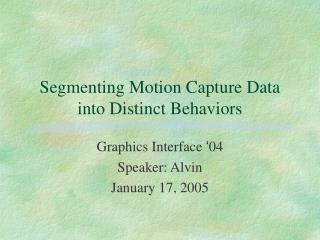Download PresentationSegmenting Motion Capture Data into Distinct BehaviorsSegmenting Motion Capture Data into Distinct Behaviors - PowerPoint PPT Presentation

Download PresentationSegmenting Motion Capture Data into Distinct Behaviors
An Image/Link below is provided (as is) to download presentation

Download Policy: Content on the Website is provided to you AS IS for your information and personal use and may not be sold / licensed / shared on other websites without getting consent from its author. While downloading, if for some reason you are not able to download a presentation, the publisher may have deleted the file from their server.

- - - - - - - - - - - - - - - - - - - - - - - - - - - E N D - - - - - - - - - - - - - - - - - - - - - - - - - - -
Presentation Transcript

1. Segmenting Motion Capture Data into Distinct Behaviors Graphics Interface ‘04 Speaker: Alvin January 17, 2005

2. Outline • Introduction • Related Work • PCA • PPCA • GMM • Results • Conclusions Segmenting Motion Capture Data into Distinct Behaviors

3. Introduction • Motion data are segmented at capture or by hand and are often small clips. • Longer shots contain natural transitions. • Segment motion into high-level behaviors. • Unsupervised Learning • Focus on efficient techniques: PCA, PPCA and GMM. Segmenting Motion Capture Data into Distinct Behaviors

4. Related Work • Model-based Approach • Low-level • Detect zero crossings of angular velocities. • Motion texton • State Machine or Motion Graph • High-level • HMM • Clustering Segmenting Motion Capture Data into Distinct Behaviors

5. Goal • Input: Motion data (14 motions, each 8000 frames) • FPS=120 • 14 Joints • Specify the rotation relative to the parent for all joints. • Rotations are specified by quaternions. • Output: Motion Clips • Automatically • Distinct Behaviors • Longer Segmenting Motion Capture Data into Distinct Behaviors

6. PCA Center of motion: Approximation: SVD: Dimension: Projection Error: Derivative: Segmenting Motion Capture Data into Distinct Behaviors

7. PCA Cut if di more than 3 standard deviations from the average Segmenting Motion Capture Data into Distinct Behaviors

8. Probabilistic PCA • Average square of discard singular values: • Covariance Matrix: • Average Mahalanobis Distance • T=150, K=T • K:=K+△, △=10, Threshold R=15 Segmenting Motion Capture Data into Distinct Behaviors

9. PPCA Segmenting Motion Capture Data into Distinct Behaviors

10. Gaussian Mixture Model • Pre-processing: • Use PCA to project onto lower dimensional subspace. (Speed up EM) • Preserve 90% of the variance. • Each cluster is represented by a Gaussian Distribution. • EM • Estimate mean, covariance matrix, prior Segmenting Motion Capture Data into Distinct Behaviors

11. GMM Segmenting Motion Capture Data into Distinct Behaviors

12. GMM Cut if frames xi and xi+1 belong to different clusters Segmenting Motion Capture Data into Distinct Behaviors

13. Results Error Matrix for PPCA Error Matrix for PCA Segmenting Motion Capture Data into Distinct Behaviors

14. Results Segmenting Motion Capture Data into Distinct Behaviors

15. Results Precision: Reported correct cuts / The total number of reported cuts Recall : Reported correct cuts / The total number of correct cuts Segmenting Motion Capture Data into Distinct Behaviors

16. 論文簡報部份 完整性介紹(4) 系統性介紹(4) 表達能力(3) 投影片製作(3) 論文審閱部分 瞭解論文內容(4) 結果正確性與完整性 (4) 原創性與重要性(4) 讀後啟發與應用： Evaluation Form The mahalanobis distance can be adopted to my classification of motions. Besides, maybe I can exploit the GMM technique to classify for comparison. Segmenting Motion Capture Data into Distinct Behaviors

17. Conclusions • Imperfect because observations’ opinions. • Treat all weights of DOF equally. • Each method require some parameters. • PCA-based methods work well. • ICA may achieve better cut detection. • No segmentation will apply for all applications. Segmenting Motion Capture Data into Distinct Behaviors

18. Mahalanobis Distance • Dt(x) = (x–mt)S-1t(x–mt)' • Dt is the distance from t group • St represents the within-group covariance matrix • mt is the vector of the means of t group • X is the vector of frame values at location x • Superior to Euclidean distance because it takes distribution of the points (correlations) into account • Useful to determine the ”similarity” from an unknown sample to known samples • Classify observations into different groups Segmenting Motion Capture Data into Distinct Behaviors

19. GMM by Using EM Segmenting Motion Capture Data into Distinct Behaviors